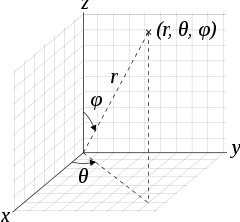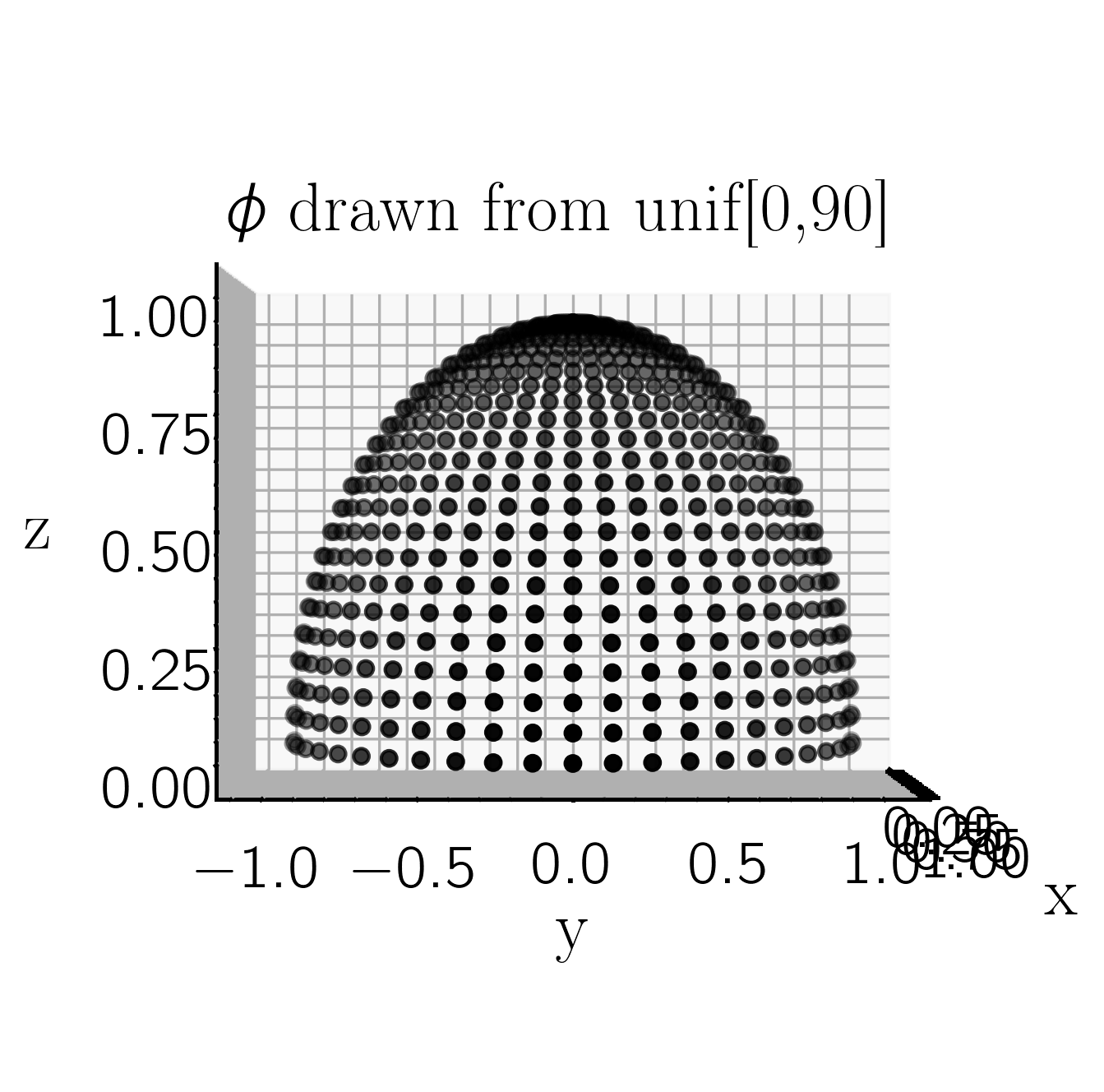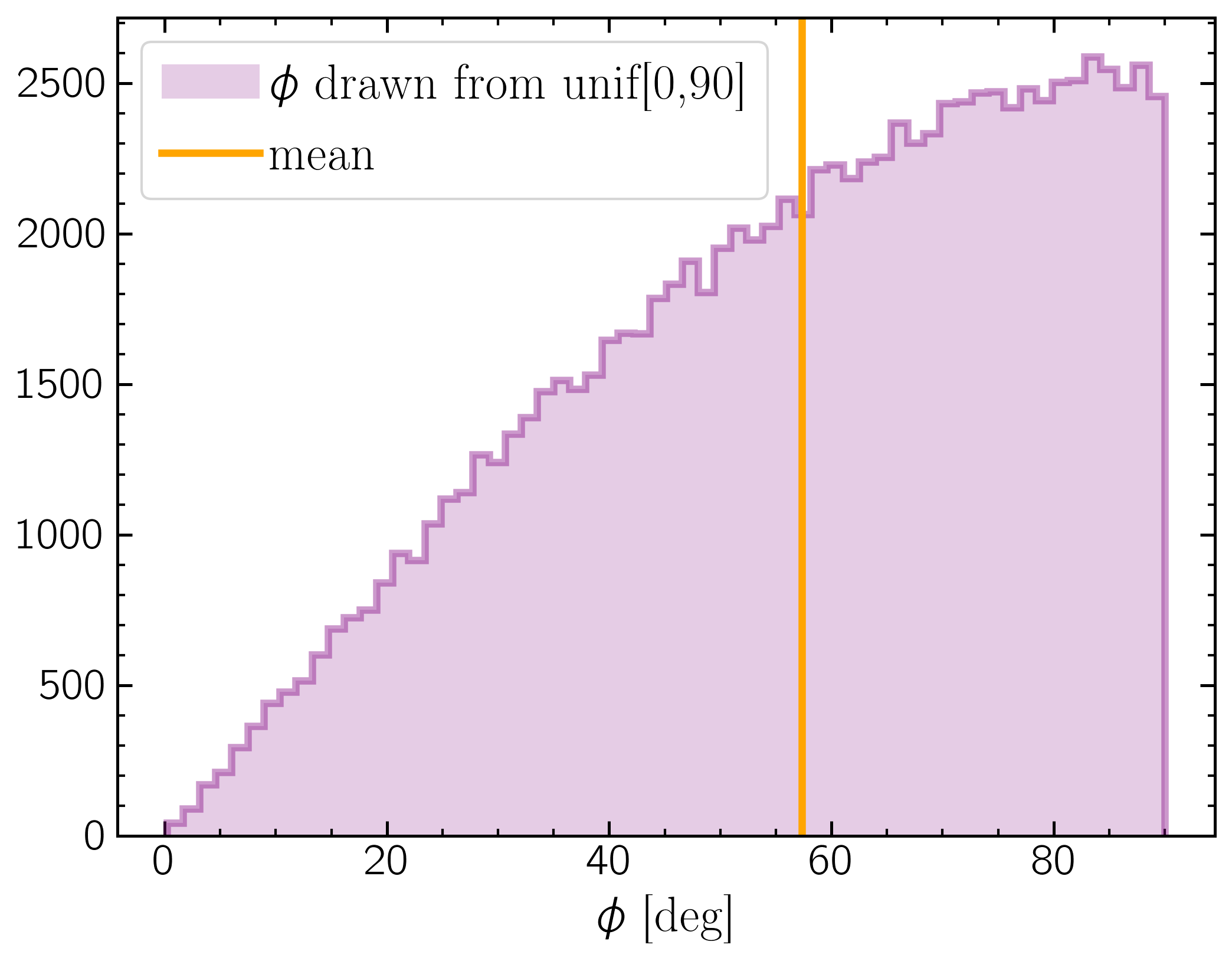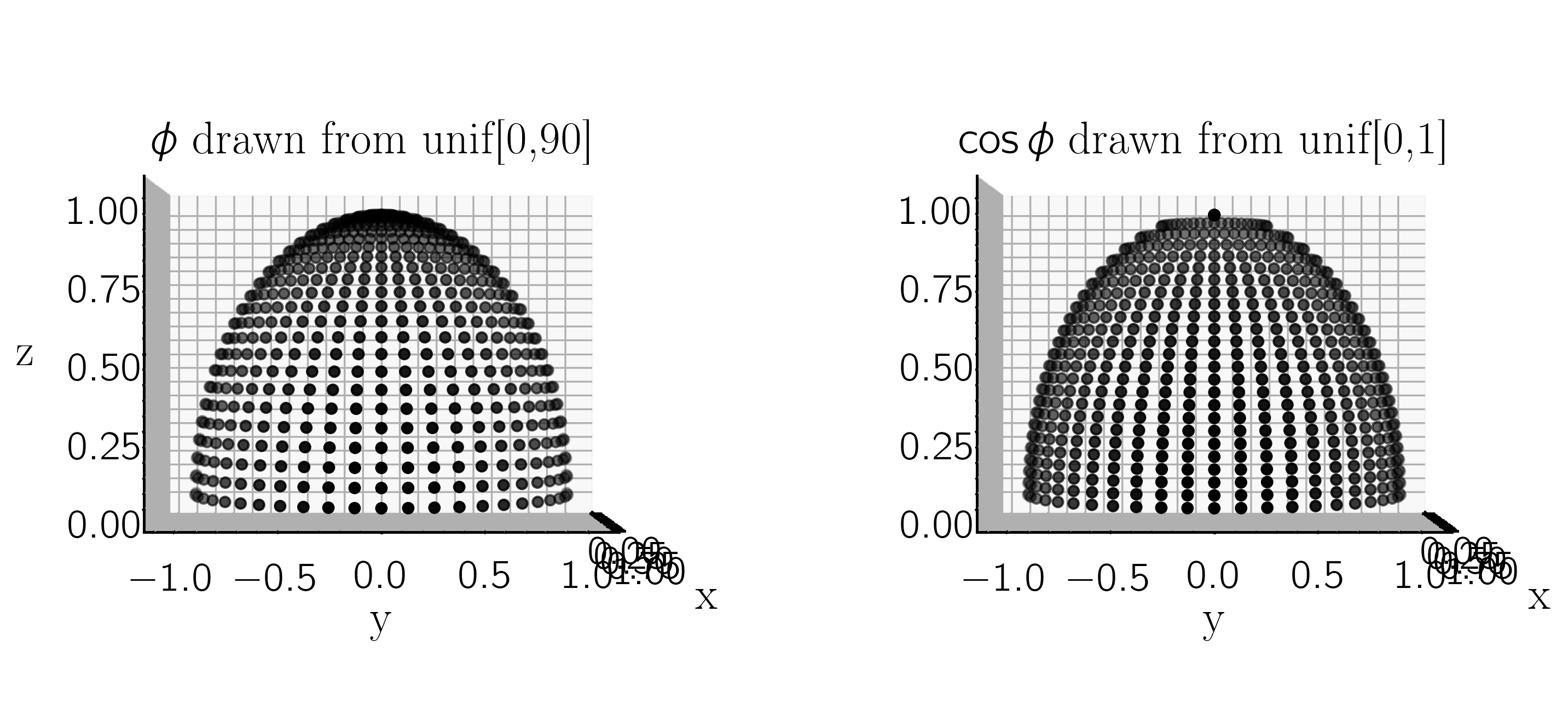## Why is 60 deg the average inclination for a uniform half-sphere?

In a spherical polar coordinate system:where$\theta = \tan^{-1} \frac{y}{x}$, $\phi = \cos^{-1} \frac{z}{r}$, $r = [x^2 + y^2 + z^2]^{1/2}$.

When viewing a Keplerian orbit projected onto the plane of the sky, where the sky plane is the ($y$,$z$) plane and $+x$ is towards the observer, then orbital inclination is the same as $\phi$ in this system. Inclination is symetrical about the $y$-axis, with incl $<$90$^{o}$ corresponding to a prograde or counter-clockwise orbit, and incl $>$90$^{o}$ being retrograde or clockwise.
So, considering a unit half-sphere in this coordinate system, with $r = 1$, $\theta$ [-90, 90], and $\phi$ [0,90] (or $x$ [-1,1], $y$ [-1,1], and $z$ [0,1]), if we draw values of $\phi$ and $\theta$ from uniform distributions on those intervals (uniformly sampling the sphere), we get a sampling of the projected uniform sphere that looks likeBecause of the projection onto the ($y,z$) plane, points near $\phi$ = 90 are more sparsely sampling the projected sphere than points near $\phi$ = 0. Looking at the histogram of points, it's clear that $\phi$ = 60$^{o}$ is the mean value.### Mathematically

The average of a function is given by $$< f > \;=\; \frac{1}{b - a} \int_{a}^{b} f(x) \,dx$$ Thus $$< \phi > \;=\; \frac{1}{1 - 0} \int_{1}^{0} \arccos(z) \,dz$$ We must use integration by parts to solve:
 $$\begin{array}{rcll} \int u\; dv & = & u\,v - \int v\; du \\ u & = & \arccos(z) \\ dv & = & dz \\ du & = & \frac{-1}{\sqrt{1 - z^2}}\; dz \\ v & = & z \\ \int_{1}^{0} \arccos(z) \,dz & = & z\; \arccos(z) \bigg\rvert_{0}^{1} - \int_{0}{1} \frac{-z}{\sqrt{1 - z^2}} dz\\ & = & \left[(1\times 0) - (0\times 1])\right] + \int_{0}^{1} \frac{-z}{\sqrt{1 - z^2}} dz\\ w & = & 1 - z^2\\ dw & = & -2z\; dz\\ & = & \int_{0}{1} \frac{1/2\; dw}{\sqrt{w}} \\ & = & \sqrt{1 - z^2} \bigg\rvert_{0}^{1} \\ & = & 1 \;\mathrm{radians}\\ & = & 57.3^{\circ} \end{array}$$

### Inclination in orbit fitting

This is why when we draw orbital parameters from prior distributions for orbit fitting (see Blunt et al. 2017, amoung many many others), we draw inclination from a distribution that is uniform in $\cos(i)$ on [-1,1] rather than uniform in $i$ on [0,180] :